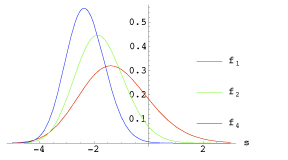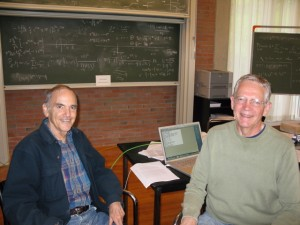# Month: May 2011

I have just read an excellent post (in French) on Freakonometrics, the remarkable blog of Arthur Charpentier.Here are some of my favorite nonlinear formulas in linear algebra. Most of them play a crucial role in mathematics such as in numerical analysis, in statistics, and in random matrix theory. In what follows, the letters ${A,B,C,D,U,V,W,M}$ denote matrices while the letters ${u,v}$ denote column vectors (special matrices).

• The blockwise inversion formula states that as soon as both sides make sense

$\begin{pmatrix} A & B \\ C & D \end{pmatrix}^{-1} = \begin{pmatrix} A^{-1}+A^{-1}B(D-CA^{-1}B)^{-1}CA^{-1} & -A^{-1}B(D-CA^{-1}B)^{-1} \\ -(D-CA^{-1}B)^{-1}CA^{-1} & (D-CA^{-1}B)^{-1} \end{pmatrix}$

The matrix ${D-CA^{-1}B}$ is the Schur complement of ${A}$ in the ${(A,B,C,D)}$ block-matrix above. In particular for every ${n\times n}$ matrix ${M}$ and any non empty ${I\subset\{1,\ldots,n\}}$ we have

$(M^{-1})_{I,I} = (M_{I,I} – M_{I,I^c}(M_{I^c ,I^c})^{-1}M_{I^c,I})^{-1} .$

The Schur complement is at the heart of the Gaussian linear model in statistics. We have already discussed the role of the Schur complement in the geometry of positive definite matrices, and an application to the computation of the Wasserstein distance between two Gaussians.

• The Woodbury matrix identity states that as soon as both sides make sense we have

$(A+UCV)^{-1}=A^{-1}-A^{-1}U(C^{-1}+VA^{-1}U)^{-1}VA^{-1}$

This contains as a special case the Sherman-Morrison formula

$(A+uv^\top)^{-1}=A^{-1}-\frac{A^{-1}uv^\top A^{-1}}{1+v^\top A^{-1}u}$

These formulas are at the heart of the Gaussian Kalman filter in engineering and the Cauchy-Stieltjes trace-resolvent method in random matrix theory

• The binomial inverse theorem provides an alternative to the Woodbury formula

$(A+UCV)^{-1} =A^{-1} – A^{-1}UC(C+CVA^{-1}UC)^{-1}CVA^{-1}$

• The matrix determinant lemma states that as soon as both sides make sense we have the identity

$\det(A+UWV^\top)=\det(W^{-1}+V^\top A^{-1}U)\det(W)\det(A)$

This contains as a special case the Sylvester determinant theorem

$\det(I_n+AB)=\det(I_m+BA)$

where ${I_d}$ is the ${d\times d}$ identity matrix, and also the formula

$\det(A+uv^\top)=(1+v^\top A^{-1}u)\det(A).$

• The resolvent formula states that for any square matrices ${A}$ and ${B}$ and any ${z\not\in\mathrm{spec}(A)\cup\mathrm{spec}(B)}$,

$(A-zI)^{-1}-(B-zI)^{-1} = (A-zI)^{-1}(B-A)(B-zI)^{-1}.$

It follows immediatly from the identity ${(B-zI)-(A-zI)=B-A}$.

If you don’t know these formulas, they know you (everywhere in numerical software packages).

Last Updated on 2012-02-02The Fisher-Tippet-Gnedenko theorem of classical extreme values theory tells us that if ${X_1,\ldots,X_n}$ are independent and identically distributed real random variables with common law ${L}$ then their maximum ${M_n=\max(X_1,\ldots,X_n)}$ can only converge as ${n\rightarrow\infty}$ up to translation and dilation to a Dirac mass or to one of the following three possible limiting distributions:

• Gumbel law (e.g. when ${L}$ is exponential or Gaussian)
• Fréchet law (e.g. when ${L}$ is heavy tailed)
• Weibull law (e.g. when ${L}$ has bounded support)

The attraction domains of each possible limiting distribution can be described in terms of the behavior of ${L}$ at the right hand side of its support. Intuitively, one can clearly guess the presence of a non homogeneous coin tossing game (related to the quantiles) in the records process. The Gumbel, Fréchet, and Weibull laws are the only max-stable laws. They play for the maximum the role played by stable laws for the addition.

Beyond the i.i.d. case, it turns out that the presence of repulsion or attraction between the random variables ${X_1,\ldots,X_n}$ may completely change the asymptotic fluctuations of the maximum and give rise to new distributions at the limit. A well known example is when ${X_1,\ldots,X_n}$ are the eigenvalues of a single ${n\times n}$ random Hermitian matrix drawn from the Gaussian Unitary Ensemble (GUE). In this case the asymptotic fluctuation of the maximum is given by a Tracy and Widom distribution. Beyond random matrix models, the Tracy-Widom distributions appear in the asymptotic fluctuations of several remarkable models such as:

• Longest increasing subsequence in random uniform permutations
• Last passage percolation with geometric or exponential weights in dimension ${2}$
• Polynuclear growth model (PNG)
• Geometric or exponential corner growth model
• Asymmetric simple exclusion processes (ASEP)

Each of these models allows a ridig analysis involving determinants giving rise to the Tracy-Widom law (or is related via a rigid correspondence to another model such as ASEP ${\leftrightarrow}$ Corner growth model).

It is quite natural to believe that the Tracy-Widom fluctuation will remain valid beyond the special cases above. One may think about, for instance, the last passage percolation for a large class of weights law (not only geometric or exponential). For random matrices, the universality of the Tracy-Widom fluctuations can be obtained by using various methods developped by Soshnikov, Tao-Vu, Erdos-Schlein-Yau, etc. The method used by Tao and Vu, particularly robust and versatile, is built on the Lindeberg replacement principle.

Many other stochastic models coming from combinatorial optimization or statistical physics are still waiting for a rigorous analysis. In many cases, the first order asymptotic (up to a constant) is already known by using standard techniques such as sub-additivity, Poissonization, and concentration (we have already discussed this in French for the longest increasing subsequence). In combinatorial optimization, we often start from i.i.d. points in some space and consider a functional of them. In this context, the asymptotic fluctuation for the minimum spanning tree (MST) and the minimum matching is Gaussian, while the asymptotic fluctuation in the traveling salesman problem (TSP) is not known.

The longest increasing subsequence in a sequence ${U_1,\ldots,U_n}$ of i.i.d. random uniform variables on ${[0,1]}$ corresponds to the longest increasing subsequence in a uniform permutation. If we allow some dependence between ${U_1,\ldots,U_n}$ (for instance repulsion or attraction !) then the permutation is no longer uniform and the behavior is completely open. Another interesting question is the study of the longest increasing subsequence when the permutation follows an Ewens distribution with an arbitrary temperature on the symmetric group.

Note. This post is partly inspired from informal discussions with the participants of École de Physique des Houches following a course by Kurt Johansson. One can regret the absence of a book on this beautiful subject. You will find nice pictures and java applets on the personal page of Patrik Ferrari.Syntax · Style · .